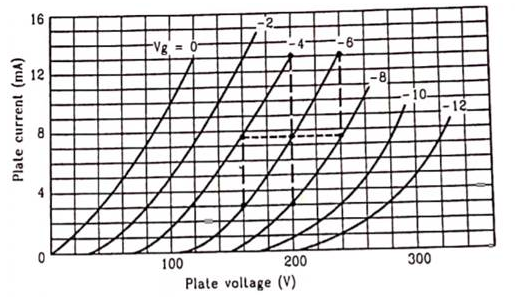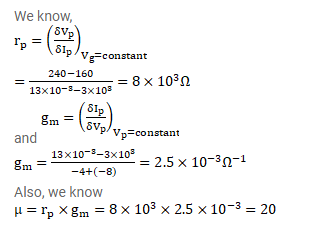# Find the values of rp, μ and gm of a triode operating at plate voltage 200V and

Question:

Find the values of $r_{p}, \mu$ and $g_{m}$ of a triode operating at plate voltage $200 \mathrm{~V}$ and grid voltage $-6 \mathrm{~V}$. The plate characteristics as shown in figure.Solution: